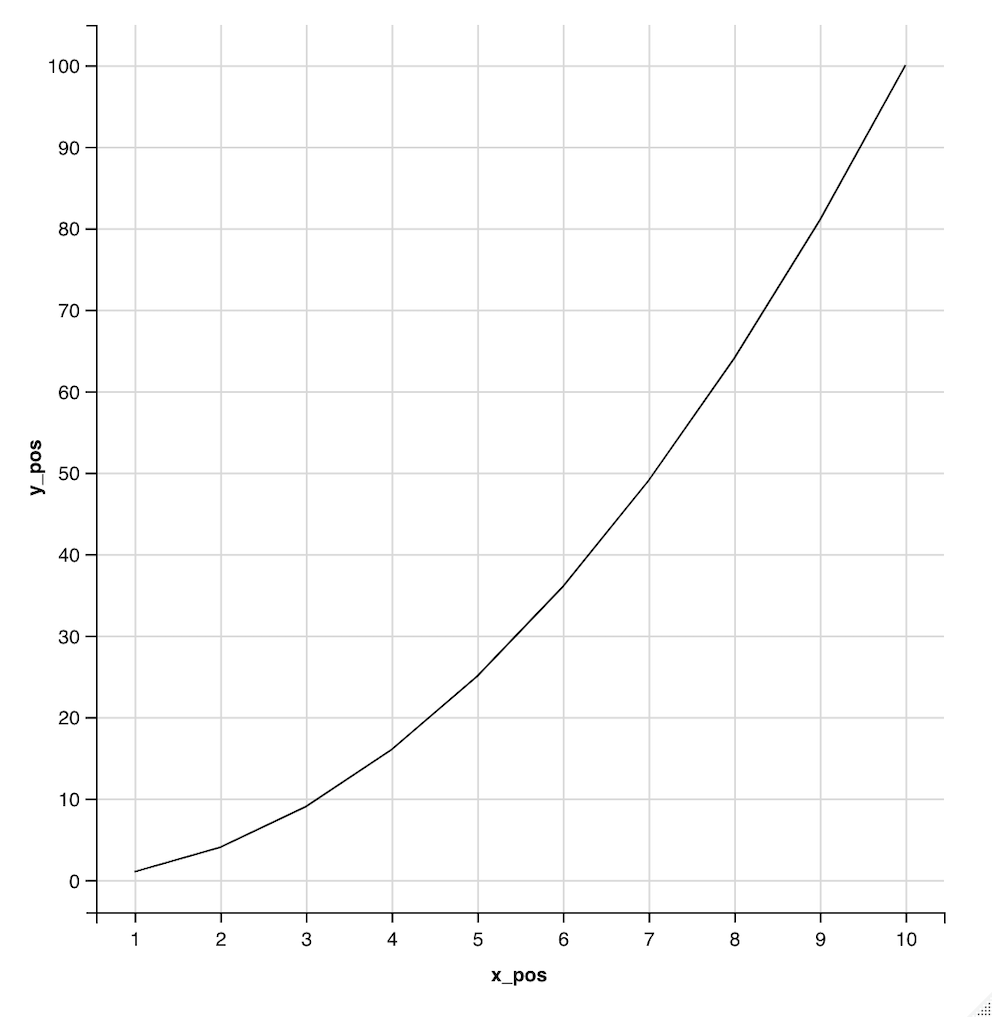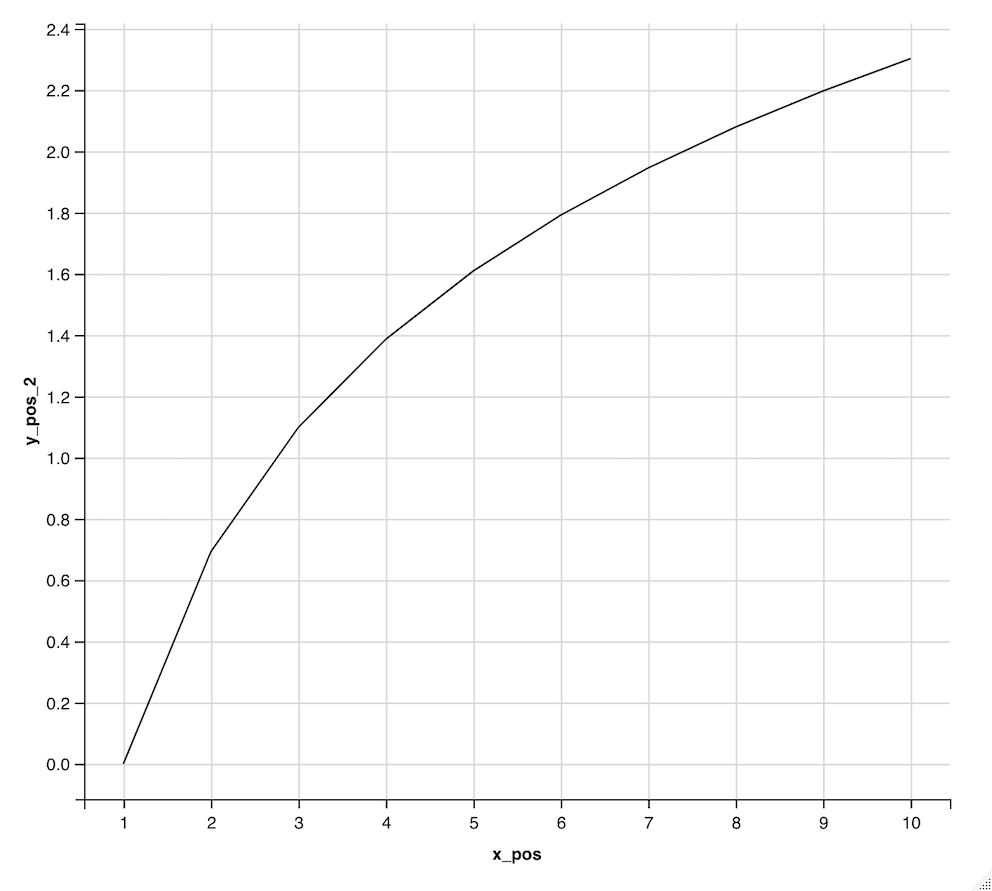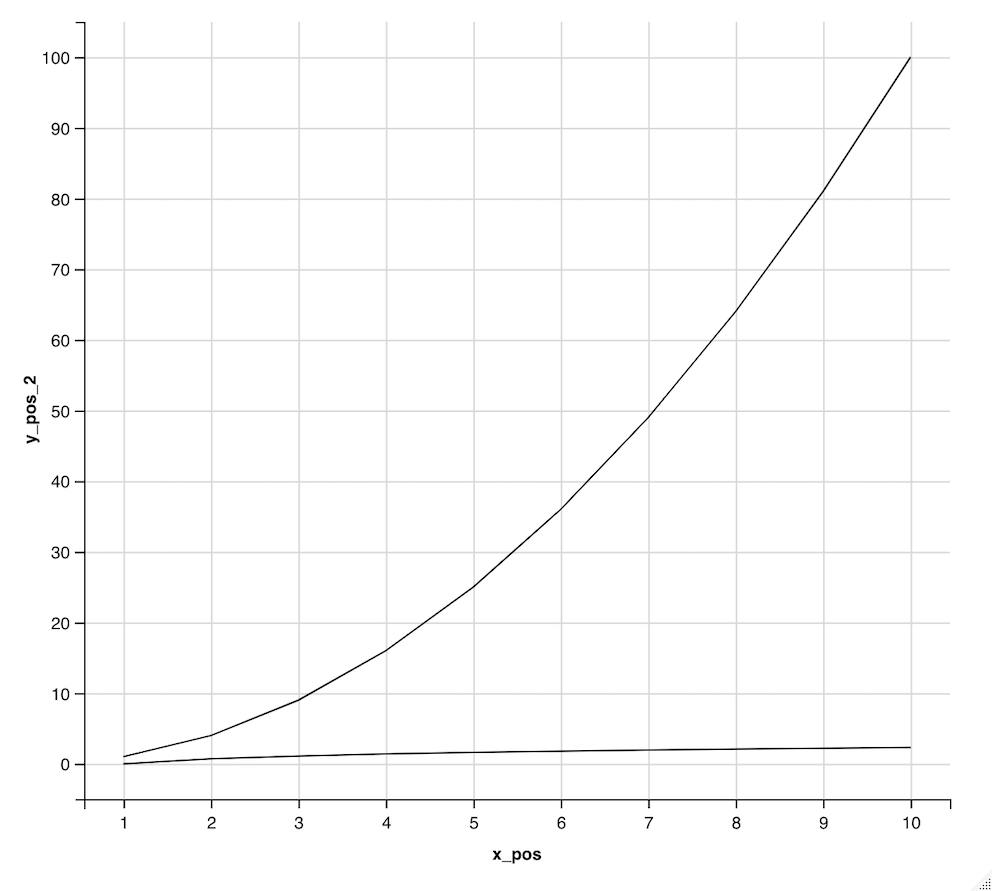Open in App
Not now

# How To Put Multiple Graphs In One Plot With Ggvis in R

• Last Updated : 02 Aug, 2022

In this article, we will be looking at the approach to putting multiple graphs in one plot using ggvis in the R programming language.

The ggplot2 library in R is used to make a graphical representation of the data provided. The package can be downloaded and installed into the working space using the following command :

`install.packages("ggplot2")`

A data frame is initially created using the data.frame() method. The data points are constructed in the data frame. The data frame is then subjected to the ggvis operations using the pipe operator. The ggvis method is used to start ggvis graphical window. The ggvis method has the following syntax :

ggvis( data , mp1, mp2.,)

Arguments :

• data – The dataset to plot
• mp1, mp2,.. – The map variables to plot

The layer_paths() method can be used to add a layer to the existing ggvis plot. It is used to represent the specified points in the form of a line segment on the plot.

## R

 `# installing the required packages  ` `library``(``"ggplot2"``) ` `library``(``"ggvis"``) ` ` `  `# creating data frame1  ` `x_pos = 1:10 ` `y_pos = x_pos^2 ` ` `  `df1 = ``data.frame``(x_pos,y_pos) ` `print``(``"Data Frame1"``) ` `print``(df1) ` ` `  `# plotting the ggvis plot1  ` `df1 %>% ``ggvis``(~x_pos,~y_pos) %>% ``layer_paths``()`

Output

``` "Data Frame1"
x_pos y_pos
1      1     1
2      2     4
3      3     9
4      4    16
5      5    25
6      6    36
7      7    49
8      8    64
9      9    81
10    10   100```Plot 1 constructed between x and x^2

Another plot is constructed using the ggvis() method between the points x and log x.

## R

 `# installing the required packages  ` `library``(``"ggplot2"``) ` `library``(``"ggvis"``) ` ` `  `# creating data frame1  ` `x_pos = 1:10 ` `y_pos_2 = ``log``(x_pos) ` `df2 = ``data.frame``(x_pos,y_pos_2) ` `print``(``"Data Frame2"``) ` `print``(df2) ` `df2 %>% ``ggvis``(~x_pos,~y_pos_2) %>% ``layer_paths``()`

Output

``` "Data Frame2"
x_pos   y_pos_2
1      1 0.0000000
2      2 0.6931472
3      3 1.0986123
4      4 1.3862944
5      5 1.6094379
6      6 1.7917595
7      7 1.9459101
8      8 2.0794415
9      9 2.1972246
10    10 2.3025851```Plot 2 constructed between x and log x

The layer_paths() method can be used to add layer to the existing plot. It can be used to add line segments to the plot. As a result, multiple line segments can be added to a single plot.

layer_paths(x = , y = ), where x and y are x and y axes respectively

The below code snippet illustrates the following procedure for combining the plots in R. Initially, a data frame is created with three columns, the first one having a sequence of elements from 1 to 10. The second one is an expression with the square of column 1 and column 3 containing the log of the first column value. The ggvis() method is then applied to construct a plot using the first two columns of the data frame. The line is added using the layer_paths() method. Another layer in the form of a line segment can then be added using the first and third columns of the data frame. Both the line segments can be added to the same plot.

## R

 `# installing the required packages  ` `library``(``"ggplot2"``) ` `library``(``"ggvis"``) ` ` `  `# creating data frame1  ` `x_pos = 1:10 ` `y_pos = x_pos^2 ` `y_pos_2 = ``log``(x_pos) ` ` `  `# creating the data frame ` `df1 = ``data.frame``(x_pos,y_pos, y_pos_2) ` `print``(``"Data Frame1"``) ` `print``(df1) ` ` `  `# plotting the data for two different axes ` `df1 %>% ``ggvis``(~x_pos,~y_pos) %>% ``layer_paths``()%>% ` `       ``layer_paths``(x = ~x_pos, y = ~y_pos_2)`

Output

``` "Data Frame1"
> print(df1)
x_pos y_pos   y_pos_2
1      1     1 0.0000000
2      2     4 0.6931472
3      3     9 1.0986123
4      4    16 1.3862944
5      5    25 1.6094379
6      6    36 1.7917595
7      7    49 1.9459101
8      8    64 2.0794415
9      9    81 2.1972246
10    10   100 2.302585```My Personal Notes arrow_drop_up
Related Articles